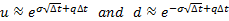/

### Enterprise Risk Management Formula Book 8. Financial derivatives

8.1          Forward prices

The no arbitrage (fair) forward price which parties should agree to exchange a security at time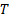if it is priced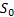now and is entitled to fixed income of present value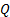in the meantime is: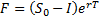whereis the interest rate (continuously compounded).

If instead it pays dividends at a rate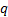(continuously compounded) then the forward prices is: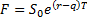8.2          Black-Scholes formulae

Geometric Brownian motion for a security (stock) price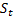involves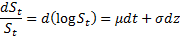The partial differential equation satisfied by values of payoffs involving such security prices is: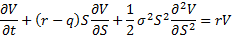whereis the interest rate,is the dividend yield (both continuously compounded) and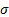is the security price volatility.

Garman-Kohlhagen formulae for values at timeof European-style put and call options with strike price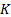maturing at time:

 Call option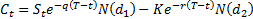Put option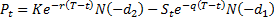where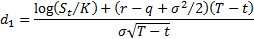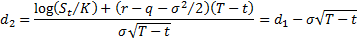We then have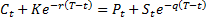, i.e. put-call parity.

Technically the Black-Scholes formulae are special cases of the Garman-Kohlhagen formulae for stocks that pay no dividend, i.e. have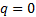, although in practice the two names are normally treated as interchangeable.

The Black-Scholes option pricing formulae can also be derived as the limit of binomial trees (lattices) with movements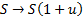or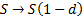with an up-step probability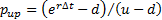and a down-step probability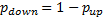where: